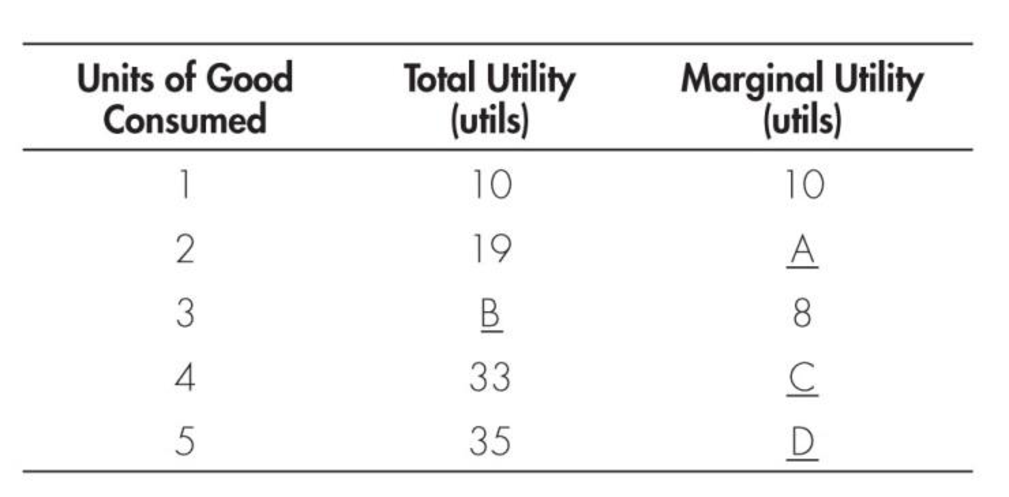Chapter 7, Problem 2WNG

Chapter
Section
Textbook Problem

Fill in blanks A–D in the following table.To determine

Identify the values of total utility and the marginal utility.

Explanation

Marginal utility (MU) can calculated using the following formula:

Marginal utility=Change in total utilityChange in quantity (1)

Substitute the respective values from the given table in Equation-1, to get the value of MU at Point A as follows:

Marginal utility=191021=91=9

Thus, MU at Point A is 9.

The value of total utility at Point B, or at 3 units can be calculated as follows:

Total utility3units=Total utility2units+Marginal utility3units=19+8=27

Thus, at Point B, the total utility is 27

Still sussing out bartleby?

Check out a sample textbook solution.

See a sample solution

The Solution to Your Study Problems

Bartleby provides explanations to thousands of textbook problems written by our experts, many with advanced degrees!

Get Started

What is the endowment effect?

Economics (MindTap Course List)

What is a nondiscretionary bonus?

PAYROLL ACCT.,2019 ED.(LL)-TEXT

INTEREST RATE PARITY Assume that interest rate parity holds. In both the spot market and the 90-day forward mar...

Fundamentals of Financial Management, Concise Edition (with Thomson ONE - Business School Edition, 1 term (6 months) Printed Access Card) (MindTap Course List)